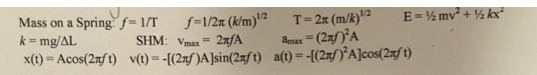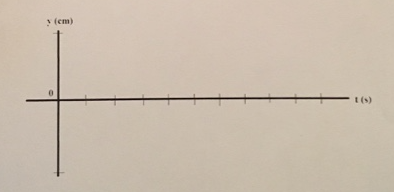# Problem: 1. The total mechanical energy of a simple harmonic oscillating system is (a) zero as it passes the equilibrium point (b) zero when it reaches the maximum displacement (c) a maximum when it passes through the equilibrium point (d) a minimum when it passes through the equilibrium point (e) a non-zero constant. 2. A mass on a spring undergoes SHM. When the mass passes through the equilibrium position, which of the following statements about it are true'' (There could be more than one correct choice) (a) Its acceleration is zero (b) Its speed is zero (c) Its elastic potential energy is zero (d) Its kinetic energy is a maximum (e) Its total mechanical energy is zero 3. A spring has an unstretched length of 12 cm. When an 80 g ball is hung from it, the length increases by 4.0 cm. Then the ball is pulled down another 4.0 cm and released. (a) What is the spring constant of the spring? (b) What is the period of the oscillation? (c) Draw a position-vs-time graph showing the motion of the ball for three cycles of the oscillation. Let the equilibrium position of the ball be y = 0 Be sure to include appropriate units on the axes so that the period and the amplitude of the motion can be determined from your graph.

###### FREE Expert Solution

1.

As a system undergoes simple harmonic motion, the total energy keeps on changing from potential energy to kinetic energy.

97% (207 ratings)###### Problem Details1. The total mechanical energy of a simple harmonic oscillating system is

(a) zero as it passes the equilibrium point

(b) zero when it reaches the maximum displacement

(c) a maximum when it passes through the equilibrium point

(d) a minimum when it passes through the equilibrium point

(e) a non-zero constant.

2. A mass on a spring undergoes SHM. When the mass passes through the equilibrium position, which of the following statements about it are true'' (There could be more than one correct choice)

(a) Its acceleration is zero

(b) Its speed is zero

(c) Its elastic potential energy is zero

(d) Its kinetic energy is a maximum

(e) Its total mechanical energy is zero

3. A spring has an unstretched length of 12 cm. When an 80 g ball is hung from it, the length increases by 4.0 cm. Then the ball is pulled down another 4.0 cm and released.

(a) What is the spring constant of the spring?

(b) What is the period of the oscillation?

(c) Draw a position-vs-time graph showing the motion of the ball for three cycles of the oscillation. Let the equilibrium position of the ball be y = 0 Be sure to include appropriate units on the axes so that the period and the amplitude of the motion can be determined from your graph.